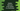# Python program to find the sum of all values of a dictionary## Python program to find the sum of all the values of a dictionary :

In this tutorial, we will learn how to find the sum of all the values of a dictionary in python.

Python dictionary is an unordered collection. It is used to store key-value pairs of data. The data is actually stored as a value in the dictionary and the key is used to indicate its corresponding value. All key-value pairs are stored inside curly braces ({}) and all items are separated by a comma.

One thing we should keep in mind that the keys must be unique in a dictionary. We can’t have the same key for two different values.

For accessing any element in a dictionary, keys are used. Using a key, we can get its corresponding value. Dictionary is mutable. We can add a new item or change an existing item in a dictionary.

Key is used to access or change any item in a dictionary. Using a key, we can also access the value associated with it. We are going to use it in this tutorial.

Our goal is to find out the sum of all values in a dictionary. We will read the values associated with each key in the dictionary and add them. For that, we will not use any loop or we are not iterating through the values. We will use one different inbuilt method to get all values on one go. The program will make everything clear to you.

The algorithm of the program is as below :

### Algorithm :

1. Create one dictionary with some key-value pairs. Alternatively, we can also take the inputs from the user. But in this example, we are creating one dictionary at the start with predefined elements.

2. Get all values of the dictionary. _‘.values()’  _is used to get the values for all keys in the dictionary. This method doesn’t take any parameter and it is an inbuilt method of python dictionary.

3. Get the sum of all values we received in step 2. _‘sum()’  _method is used to get the sum of all the values.

4. Store the sum in a variable.

5. Print out the sum.

### Python Program:

``````my_dictionary = {'1' : 1,'2' : 2,'3' : 3,'4' : 4,'5' : 5}

print("Given Dictionary : ",my_dictionary)
print("values ",my_dictionary.values())

sum_values = sum(my_dictionary.values())

print("Sum of all the values in the dictionary is : ",sum_values)``````